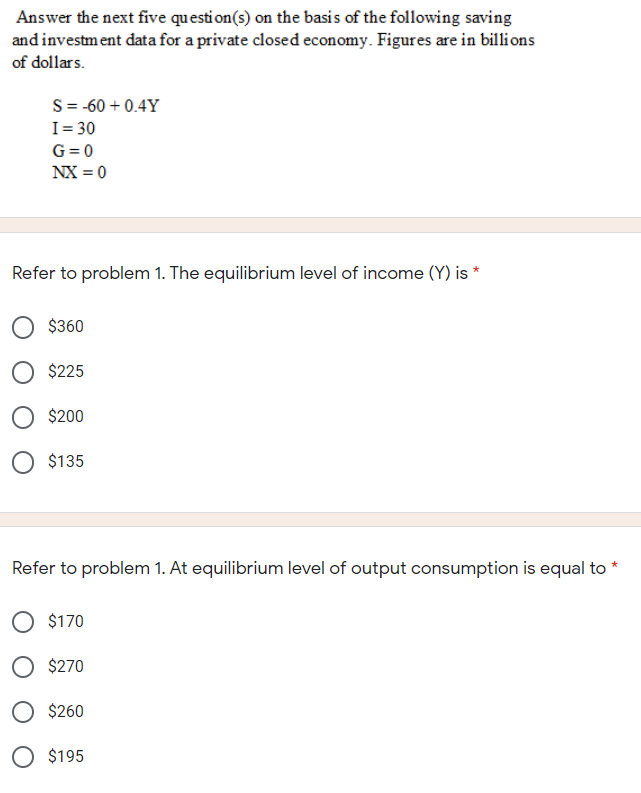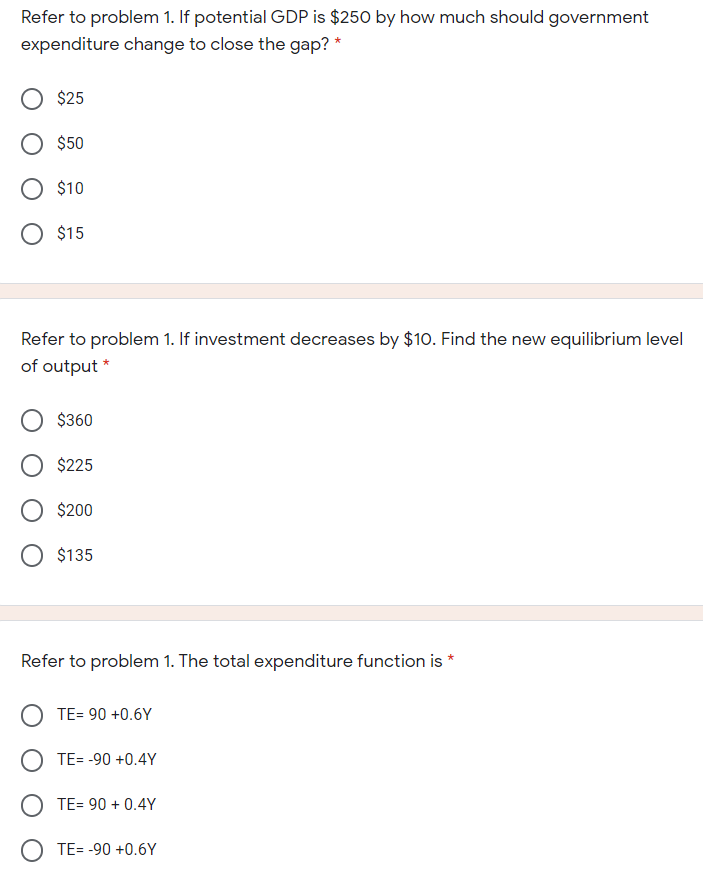### Create an Account

Already have account?

### Forgot Your Password ?

Home / Questions / Answer the next five question(s) on the basis of the following saving and investment data ...

# Answer the next five question(s) on the basis of the following saving and investment data for a private closed economy. Figures are in billions of dollars. S= -60 +0.4Y I = 30 G=0 NX = 0 Refer to prob

Answer the next five question(s) on the basis of the following saving and investment data for a private closed economy. Figures are in billions of dollars. S= -60 +0.4Y I = 30 G=0 NX = 0 Refer to problem 1. The equilibrium level of income (Y) is * \$360 O \$225 \$200 O \$135 Refer to problem 1. At equilibrium level of output consumption is equal to * O \$170 \$270 O \$260 \$195
Refer to problem 1. If potential GDP is \$250 by how much should government expenditure change to close the gap? * \$25 \$50 \$10 O \$15 Refer to problem 1. If investment decreases by \$10. Find the new equilibrium level of output * \$360 \$225 \$200 \$135 Refer to problem 1. The total expenditure function is * TE= 90 +0.6Y OTE=-90 +0.4Y TE= 90 + 0.4Y OTE= -90 +0.6YApr 15 2021 View more View Less

#### Answer (Solved)Subscribe To Get Solution